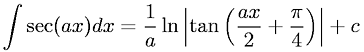Equations > Calculus > Integration/Integrals > Integral of secant

### Integral of secantLatex Code:

MathML Code:

 $\int \mathrm{sec}\left(\mathrm{ax}\right)\mathrm{dx}=\frac1a\mathrm{ln}|\mathrm{tan}\left(\frac\mathrm{ax}2+\frac\pi 4\right)|+c$

MathType 5.0: